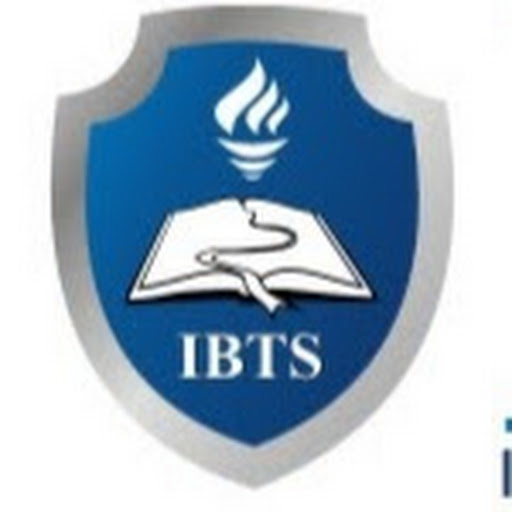# Quant for SBI Clerk/NABARD 2018: 11 April

## Quant for SBI Clerk/NABARD 2018: 11 April

1. A tank is in the shape of a cuboid whose length is 8.4m and breadth is 3.5m. Water flows into it through a pipe at the rate of 14 metres per second. The cross-section of the pipe is 7 cm × 5 cm. Find the height up to which water level will rise in the tank in 60 minutes.
1) 6m                     2) 7m                     3) 6.5m                  4) 14m                   5) 10m

2. 65% of a number is 460 more than 45% of the same number. What is 40% of that number?
1) 940                    2) 820                    3) 930                    4) 960                    5) 920

3. The difference between the simple interest and the compound interest at the rate of 10% at the end of 3 years is Rs. 620. What is the sum?
1) Rs. 20000        2) Rs. 24000        3) Rs. 40000        4) Rs. 30000        5) Rs. 35000

4. In how many different ways can the letters of the word INSPIRATIONAL be arranged so that the vowels always come together?
1) 142820             2) 145320             3) 151200             4) 151000             5) 1209600

5. A 440-metre-long train running at a speed of 36 kmph will take how much time to cross another 360-metre-long train running in the opposite direction at a speed of 44 kmph?
1) 42 seconds       2) 36 seconds       3) 25 seconds       4) 20 seconds       5) 30 seconds

6. A person bought two Karizma bikes for Rs. 185000. He sold one of them at a profit of 15% and another at a profit of 60%. He found that the selling price of both the bikes were equal. What are the cost prices of either of the bikes respectively? (Calculate the approx value.)
1) Rs. 107636, Rs. 77363                2) Rs. 107325, Rs. 77636                3) Rs. 107272, Rs. 72636
4) Rs. 108325, Rs. 77663                5) None of these

Directions (Q. 7-11): Study the following pie-chart and table carefully to answer the given questions.

7. What is the ratio of the number of female students in Institute F to that of male students in Institute C?
1) 1 : 6                   2) 2 : 3                   3) 1 : 4                   4) 6 : 1                   5) None of these

8. The total number of students studying in Institute F is what per cent of the total number of students studying in Institute C?
1) 125%                 2) 175%                 3) 225%                 4) 150%                 5) None of these

9. Which institute has the maximum number of students and which has the minimum number of students respectively?
1) E and G             2) F and C             3) B and E             4) A and E             5) E and C

10. The number of female students from Institute E is what per cent of the total number of students from all the institutes? (Calculate the approximate value.)
1) 9%                     2) 8%                     3) 7%                     4) 10%                   5) 13%

11. What is the difference between the total number of students from all the institutes and the number of male students from Institute A, D and G?
1) 46000               2) 46520               3) 46550               4) 45320               5) 46250

1. 1
2. 5
3. 1
4. 5
5. 2
6. 1
7. 5
8. 3
9. 5
10. 5
11. 4
###### Share To:### IBTS INDIA

IBTS - (Institute for Banking Training & Educational Services) is in the Leading Institution for banking & SSC, IBPS, Center & State exams in Chandigarh.